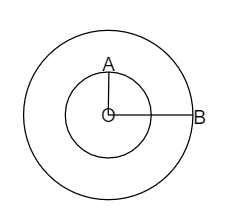# With the same centre O draw circles of radius $4\ cm$ and $2.5\ cm$.

Given:

The radii of the circles are 4 cm and 2.5 cm. The centre of the circle is O.

To do:

We have to draw two concentric circles with the given radii.

Solution:

Steps for construction:Step 1:

Take a ruler of unit length 1 cm.

Step 2 :

Take any point O and mark it as the centre.

Step 3 :

With O as centre draw a circle of radius $OA = 2.5\ cm$

Step 4 :

With O as centre draw a circle of radius $OB = 4\ cm$

Thus the concentric-circles $C_1$ and $C_2$ are drawn.

Updated on: 10-Oct-2022

31 Views